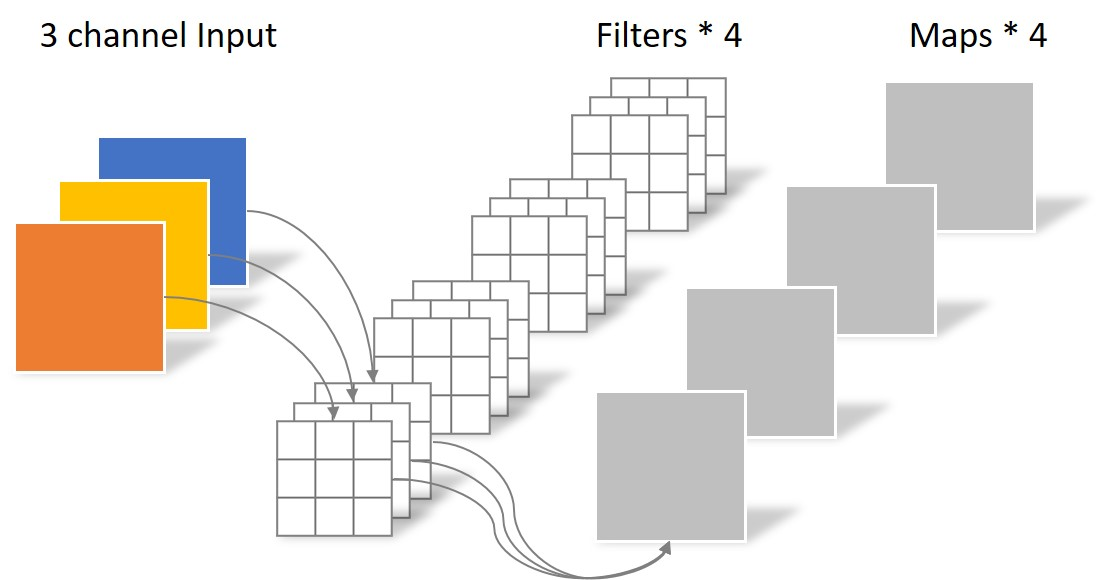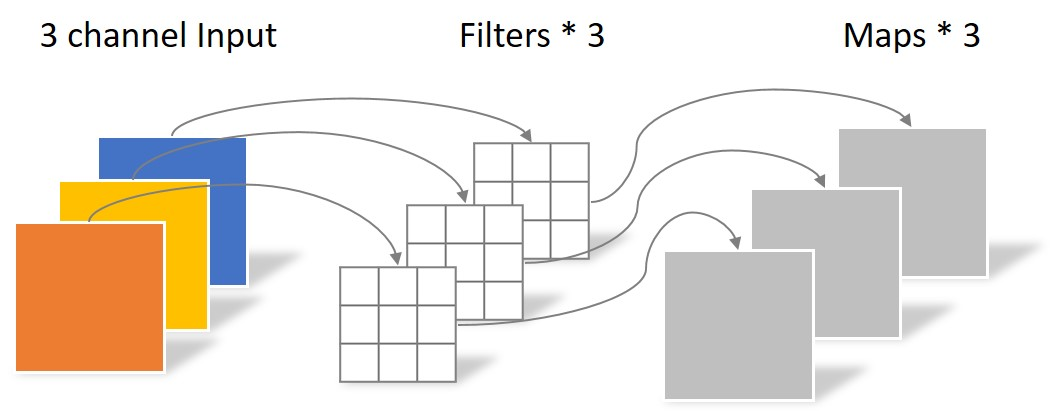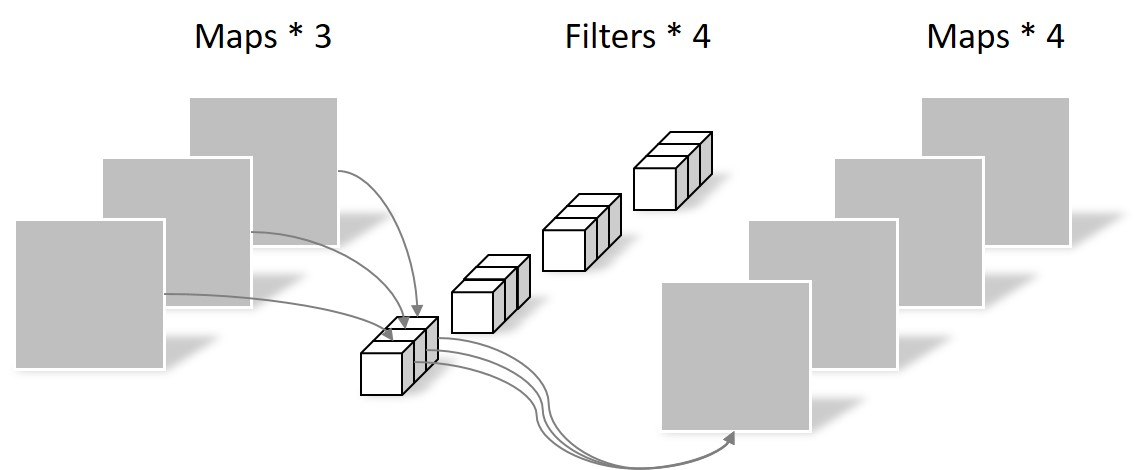# Normall convolution

approximate complexity = `out_dim` x `kernel` x `conv` = 4 x 9 x `conv`. (`conv` = `in_dim` x `in_pixels`)# Separable convolution

Separable conv = Depth-wise conv + Point-wise conv

approximate complexity = `kenel` x `conv` + `out_dim` x `conv` = (9 + 4) x `conv`. (`conv` = `in_dim` x `in_pixels`(